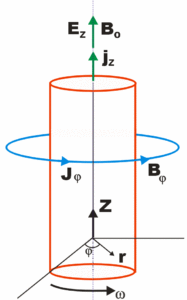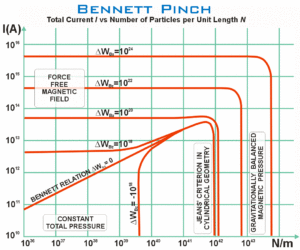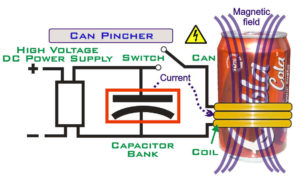# Pinch

A pinch (alternately called a “knot,” “Bennett pinch” (after Willard Harrison Bennett), “electromagnetic pinch”, “magnetic pinch”, the “pinch effect” or “plasma pinch”) is the compression of an electrically conducting filament by magnetic forces. The conductor is usually a plasma, but could also be a solid or liquid metal. In a z-pinch, the current is axial (in the z direction in a cylindrical coordinate system) and the magnetic field azimuthal; in a theta-pinch, the current is azimuthal (in the theta direction in cylindrical coordinates) and the magnetic field is axial.

Pinches occur naturally in electrical discharges such as lightning bolts, the aurora, current sheets, and solar flares. They are also produced in the laboratory, primarily for research into fusion power, but also by hobbyists.

## Pinch production and types

Pinches are created in the laboratory in equipment related to nuclear fusion, such as the Z-pinch machine and high-energy physics, such as the dense plasma focus. Pinches may also become unstable, and generate radiation across the electromagnetic spectrum, including radio waves, x-rays and gamma rays, and also neutrons and synchrotron radiation. Types of pinches, that may differ in geometry and operating forces, include the Cylindrical pinch, Inverse pinch, Orthogonal pinch effect, Reversed field pinch, Sheet pinch, Screw pinch (also called stabalized z-pinch, or θ-z pinch), Theta pinch (or thetatron), Toroidal pinch, Ware pinch , fountain pinch, and Z-pinch.

Pinches are used to generate X-rays, and the intense magnetic fields generated are used in electromagnetic forming of metals (they have been demonstrated in crushing aluminium soft drinks cans). They have applications to particle beams including particle beam weapons, and astrophysics.

## HistoryThe IEEE Emblem of the Institute of Electrical and Electronics Engineers shows the basic features of an azimuthal magnetic pinch (depicted by the arc-shaped arrow), and a vertical current depicted by the vertical arrow (in the Z-direction)The first creation of a z-pinch in the laboratory may have occurred in 1790 in Holland when Martinus van Marum created an explosion by discharging 100 Leyden jars into a wire. The pinch effect was first observed with a liquid metal in 1904. The phenomenon was not understood until 1905, when Pollock and Barraclough investigated a compressed and distorted length of copper tube from a lightning rod after it had been struck by lightning. Their analysis showed that the forces due to the interaction of the large current flow with its own magnetic field could have caused the compression and distortion. A similar, and apparently independent, theoretical analysis of the pinch effect in liquid metals was published by Northrupp in 1907.. The next major development was the publication in 1934 of an analysis of the radial pressure balance in a static z-pinch by Bennett (See the following section for details.)

Thereafter, the experimental and theoretical progress on pinches was driven by fusion power research. In their article on the “Wire-array z-pinch: a powerful x-ray source for ICF”, M G Haines et al, wrote on the “Early history of z-pinches”:

In 1946 Thompson and Blackman  submitted a patent for a fusion reactor based on a toroidal z-pinch  with an additional vertical magnetic field. But in 1954 Kruskal and Schwarzchild  published their theory of MHD instabilities in a z-pinch. In 1956 Kurchatov gave his famous Harwell lecture showing nonthermal neutrons and the presence of m = 0 and m = 1 instabilities in a deuterium pinch . In 1957 Pease  and Braginskii  independently predicted radiative collapse in a z-pinch under pressure balance when in hydrogen the current exceeds 1.4 MA. (The viscous rather than resistive dissipation of magnetic energy discussed above and in  would however prevent radiative collapse). Lastly, at Imperial College in 1960, led by R Latham, the Rayleigh–Taylor (RT) instability was shown, and its growth rate measured in a dynamic z-pinch .”

## Formal treatmentThe Generalized Bennett Relation considers a current-carrying magnetic-field-aligned cylindrical plasma pinch undergoing rotation at angular frequency ω

### The Bennett Relation

Consider a cylindrical column of fully ionized plasma, with an axial electric field, producing an axial current density, j, and associated azimuthal magnetic field, B. As the current flows through its own magnetic field, a pinch is generated with an inward radial force density of j x B. In a steady state with forces balancing:

p = ∇(pe + pi) = j x Β

where ∇p is the magnetic pressure gradient, pe and pi is the electron and ion pressures. Then using Maxwell’s equation ∇ x B = μ0 j and the ideal gas law p = N k T, we derive:

$$2 N k(T_e + T_i) = \frac{{\mu_0}} {4 \pi} I^2$$ (The Bennett Relation)

where N is the number of electrons per unit length along the axis, Te and Ti are the electron and ion temperatures, I is the total beam current, and k is the Boltzmann constant.

### The Generalized Bennett RelationThe Bennett pinch showing the total current (I) versus the number of particles per unit length (N). The chart illustrates four physically distinct regions. The plasma temperature is 20 K, the mean particle mass 3×10-27 kg, and ΔWBz is the excess magnetic energy per unit length due to the axial magnetic field Bz. The plasma is assumed to be non-rotational, and the kinetic pressure at the edges is much smaller than inside.

The Generalized Bennett Relation considers a current-carrying magnetic-field-aligned cylindrical plasma pinch undergoing rotation at angular frequency ω. Along the axis of the plasma cylinder flows a current density jz, resulting in a toroidal magnetίc field Βφ. Originally derived by Witalis,  the Generalized Bennett Relation results in :

$$\frac{1}{4} \frac{\partial^2 J_0}{\partial t^2} = W_{\perp k i n} + \Delta W_{E_z} + \Delta W_{B_z} + \Delta W_k – \frac{{\mu_0}} {8 \pi} I^2 (a)$$

$$.\qquad \qquad – \frac{1}{2}G\overline{m}^2 N^2 (a) + \frac{1}{2}\pi a^2 \epsilon_0 \left(E_r^2 (a) – E_\phi^2 (a) \right )$$

• where a current-carrying, magnetic-field-aligned cylindrical plasma has a radius a,
• J0 is the total moment of inertia with respect to the z axis,
• W⊥kin is the kinetic energy per unit length due to beam motion transverse to the beam axis
• WBz is the self-consistent Bz energy per unit length
• WEz is the self-consistent Ez energy per unit length
• Wk is thermokinetic energy per unit length
• I(a) is the axial current inside the radius a (r in diagram)
• N(a) is the total number of particles per unit length
• Er is the radial electric field
• Eφ is the rotational electric field

The positive terms in the equation are expansional forces while the negative terms represent beam compressional forces.

### The Carlqvist RelationPinched aluminium can, produced from a pulsed magnetic field created by rapidly discharging 2 kilojoules from a high voltage capacitor bank into a 3-turn coil of heavy gauge wire. Source: Bert Hickman, Stoneridge Engineering.

The Carlqvist Relation, published by Per Carlqvist in 1988, is a specialization of the Generalized Bennett Relation (above), for the case that the kinetic pressure is much smaller at the border of the pinch than in the inner parts. It takes the form

$$\frac{{\mu_0}} {8 \pi} I^2 (a) +\frac{1}{2}G\overline{m}^2 N^2 (a) = \Delta W_{B_z} + \Delta W_k$$

and is applicable to many space plasmas.

The Carlqvist Relation can be illustrated (see right), showing the total current (I) versus the number of particles per unit length (N) in a Bennett pinch. The chart illustrates four physically distinct regions. The plasma temperature is quite cold (Ti = Te = Tn = 20 K), containing mainly hydrogen with a mean particle mass 3×10-27 kg. The thermokinetic energy Wk >> π a2 pk(a). The curves, ΔWBz show different amounts of excess magnetic energy per unit length due to the axial magnetic field Bz. The plasma is assumed to be non-rotational, and the kinetic pressure at the edges is much smaller than inside.

Chart regions: (a) In the top-left region, the pinching force dominates. (b) Towards the bottom, outward kinetic pressures balance inwards magnetic pressure, and the total pressure is constant. (c) To the right of the vertical line ΔWBz=0, the magnetic pressures balances the gravitational pressure, and the pinching force is negligible. (d) To the left of the sloping curve ΔWBz=0, the gravitational force is negligible. Note that the chart shows a special case of the Carlqvist relation, and if it is replaced by the more general Bennett relation, then the designated regions of the chart are not valid.

Carlqvist further notes that by using the relations above, and a derivative, it is possible to describe the Bennett pinch, the Jean’s criterion (for gravitational instability , in one and two dimensions), force-free magnetic fields, gravitationally balanced magnetic pressures, and continuous transitions between these states.

## Crushing cans with the pinch effectMany high-voltage electronics enthusiasts make their own devices using pulsed power techniques to produce a theta pinch capable of crushing an aluminium soft drink can. (Warning! High-voltage electric shocks may be lethal).

An electromagnetic aluminium can crusher consists of four main components (1) A high voltage DC power supply which provides a source of electrical energy (2) A large energy discharge capacitor to accumulate the electrical energy (3) A high voltage switch or spark gap and (4) A robust coil through which the stored electrical energy can be quickly discharged in order to generate a correspondingly strong pinching magnetic field (see diagram below).

In practice, such a device is somewhat more sophisticated than the schematic diagram suggests, including electrical components that control the current in order to maximize the resulting pinch, and to ensure that the device works safely. For more details, see the notes .

Sam Barros’s can crusher cost about \$500, and uses the second largest SCR in production and a 900 Volt capacitor bank storing about 3000 Joules of energy. For a very short time, it generates a magnetic field 250,000 times the strength of the Earth’s magnetic field and a power of about 22 megawatts. 

## Trivia

Pinch-generating equipment was used in Ocean’s Eleven, where it was used to disrupt Las Vegas’s power grid just long enough for the characters to begin their heist.